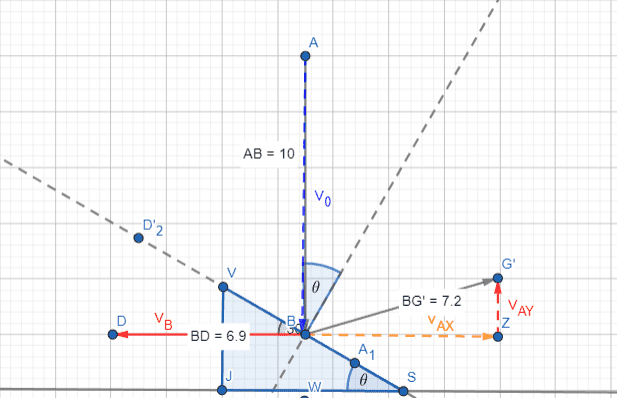# Find the magnitude and direction of the velocity

• inigooo
In summary, according to the conversation, the formula for V^2=u^2+2gh is used to find the velocity of an object after colliding with another object. The terms u, g, and h represent initial velocity, gravitational acceleration, and the height of the object, respectively. The formula for finding the magnitude and direction of the secondary ball's velocity after collision in terms of its velocity before contact is 1/2 m_1v_1^2 + 1/2 m_2v_2^2 = 1/2 m_1v_1’^2 + 1/2 m_2v_2’^2, where m represents mass and v represents velocity. Additionally,f
Case 1 limiting value would be 30∘ below the horizontal as per physics of an oblique elastic collision between 2 equal masses.
Good point. And that does validate my simulation, more or less.
could you perhaps set the string's spring constant to 100 times that of ball pair system ?
Unfortunately the simulation breaks down there. In a simple simulation of SHM, there's a tendency for the energy to grow exponentially. There are probably some standard tricks for taming this, but I haven't managed to find one.

•neilparker62
Fwiw, I have now simulated it, ignoring gravity.
For some arbitrary choices of initial ball speed, mass, etc.:
- With the string having a tenth the spring constant of the ball pair, the left hand ball loses contact with a trajectory of 30.6o below the horizontal.
- With the ball pair and string having the same spring constant, the trajectory is 21.5o below the horizontal.
- With the string having ten times the constant, the trajectory is 13.1o above the horizontal.

So as I surmised, if all is elastic, the relative spring constants are crucial.

Edit: although I intended to ignore gravity, I made the mistake of taking the string as always in tension. I need to allow it to go slack. So ignore the above results.
Having managed to tame the simulation somewhat (by taking any three consecutive values of a position or velocity coordinate to follow a quadratic) I am satisfied that when the string's spring constant is much the greater the suspended ball does not leave with any significant vertical velocity.

In the case where the string's spring constant is very high compared with that of the ball pair, I found this solution. (from page 11 of the referenced pdf). Am still trying to figure out exactly what they are doing but it looks like the limiting angle is about ##16^{\circ}## above the horizontal.

Just wondering if this situation is the same as if a ball were dropped onto a 60 degree wedge on a smooth frictionless table ? The wedge has the same mass as the ball and there is no 'slippage' on impact. Collision is elastic.

Just wondering if this situation is the same as if a ball were dropped onto a 60 degree wedge on a smooth frictionless table ? The wedge has the same mass as the ball and there is no 'slippage' on impact. Collision is elastic.
I would say it’s identical. In principle, the table surface has some elasticity and that would behave just the same as the string in the original.In the following analysis it should be noted that with respect to the Cartesian plane, ##V_0## is a negative quantity. Arbitrarily but conveniently readers may consider its value to be ##-10ms^{-1}##.

Conservation of kinetic energy: $${V_0}^2={V_B}^2+{V_{AX}}^2+{V_{AY}}^2$$
Zero net horizontal momentum: $$V_{AX}=-V_B\implies {V_0}^2=2{V_{AX}}^2+{V_{AY}}^2$$
Collision impulse(s) acting along the normal are equal and opposite ##\implies## no change in relative velocity along the normal assuming ##m_A=m_B##: $$V_0\cos\theta=V_B\sin\theta-V_{AY}\cos\theta-V_{AX}\sin\theta=-V_{AY}\cos\theta-2V_{AX}\sin\theta$$ $$\implies V_{AY}=-V_0-2V_{AX}\tan\theta$$ $$\implies {V_{AY}}^2={V_0}^2 + 4{V_{AX}}^2\tan^2\theta+4V_0V_{AX}\tan\theta$$ Substituting for ##V_B## and ##V_{AY}## in the kinetic energy equation, we obtain: $$2{V_{AX}}^2+4{V_{AX}}^2\tan^2\theta+4V_0V_{AX}\tan\theta=0$$ $$\implies V_{AX}=\frac{-2V_0\tan\theta}{1+2\tan^2\theta};V_{AY}=-V_0\frac{1-2\tan^2\theta}{1+2\tan^2\theta}$$ $$\tan\beta=\frac{1-2\tan^2\theta}{2\tan\theta}$$ where ##\beta## is the angle between the post collision trajectory of ball A and the horizontal axis.